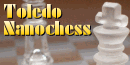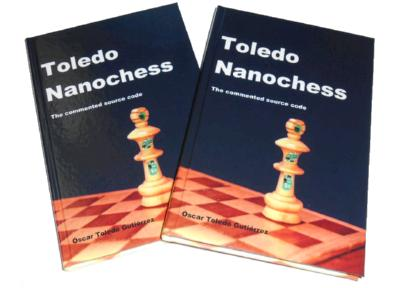# Toledo Nanochess

I've improved the Toledo Chess 1 engine to squeeze out every character possible. This reduced version was used as the basis for Toledo Chess 2, a chess program with graphical interface that can be printed on a single sheet of paper!.
This latter engine brought two branches: One devoted to create the most small chess program possible without en passant, castling and promotion only to queen, this is Toledo Picochess.
The other branch objective was to create the most small full-chess program with an enhanced evaluation function, this is Toledo Nanochess.
With only 1257 non-blank characters, Toledo Nanochess is the current world's smallest chess program in C language. At this date (Feb/13/2009) I'm not aware of smaller programs with this playing-strength.
The closest competitor is H.G. Muller with Micromax v1.6 in 1433 non-blank characters. Although Toledo Nanochess is smaller, it manages to beat gracefully Micromax v1.6, see game 1 and game 2. As both are deterministic programs, these are the only possible games, also I did a Nunn match (PGN format), a way to test chess programs with ten predeterminated start positions and alternating colors, so you can be sure of the real strength, the result was +5 =11 -4 with advantage for Toledo Nanochess.
```/**************************************************************************\
| Toledo Nanochess (c) Copyright 2009 Oscar Toledo G. All rights reserved  |
| 1257 non-blank characters. Evolution from my winning IOCCC 2005 entry.   |
| o Use D2D4 algebraic style for movements.  biyubi@gmail.com  Nov/20/2009 |
| o On promotion add a number for final piece (3=N, 4=B, 5=R, 6=Q)         |
| o Press Enter alone for computer to play.                                |
| o Full legal chess moves.                       http://www.nanochess.org |
| o Remove these comments to get 1326 bytes source code (*NIX end-of-line) |
\**************************************************************************/
char*l="ustvrtsuqqqqqqqqyyyyyyyy}{|~z|{}"
"   76Lsabcddcba .pknbrq  PKNBRQ ?A6J57IKJT576,+-48HLSU";
#define F getchar()&z
#define v X(0,0,0,21,
#define Z while(
#define _ ;if(
#define P return--G,y^=8,
B,i,y,u,b,I,*G=I,x=10,z=15,M=1e4;X(w,c,h,e,S,s){int t,o,L,E,d,O=e,N=-M*M,K
=78-h<<x,p,*g,n,*m,A,q,r,C,J,a=y?-x:x;y^=8;G++;d=w||s&&s>=h&&v 0,0)>M;do{_ o=I[
p=O]){q=o&z^y _ q<7){A=q--&2?8:4;C=o-9&z?q["& .\$  "]:42;do{r=I[p+=C[l]-64]_!w|p
==w){g=q|p+a-S?0:I+S _!r&(q|A<3||g)||(r+1&z^y)>9&&q|A>2){_ m=!(r-2&7))P G=O,
K;J=n=o&z;E=I[p-a]&z;t=q|E-7?n:(n+=2,6^y);Z n<=t){L=r?l[r&7]*9-189-h-q:0 _ s)L
+=(1-q?l[p/x+5]-l[O/x+5]+l[p%x+6]*-~!q-l[O%x+6]+o/16*8:!!m*9)+(q?0:!(I[p-1]^n)+
!(I[p+1]^n)+l[n&7]*9-386+!!g*99+(A<2))+!(E^y^9)_ s>h||1<s&s==h&&L>z|d){p[I]=n,O
[I]=m?*g=*m,*m=0:g?*g=0:0;L-=X(s>h|d?0:p,L-N,h+1,G,J=q|A>1?0:p,s)_!(h||s-1|B
-O|i-n|p-b|L<-M))P y^=8,u=J;J=q-1|A<7||m||!s|d|r|o<z||v 0,0)>M;O[I]=o;p[I]=r;m?
*m=*g,*g=0:g?*g=9^y:0;}_ L>N){*G=O _ s>1){_ h&&c-L<0)P L _!h)i=n,B=O,b=p;}N=L;}
n+=J||(g=I+p,m=p<O?g-3:g+2,*m<z|m[O-p]||I[p+=p-O]);}}}}Z!r&q>2||(p=O,q|A>2|o>z&
!r&&++C*--A));}}}Z++O>98?O=20:e-O);P N+M*M&&N>-K+1924|d?N:0;}main(){Z++B<121)*G
++=B/x%x<2|B%x<2?7:B/x&4?0:*l++&31;Z B=19){Z B++<99)putchar(B%x?l[B[I]|16]:x)_
x-(B=F)){i=I[B+=(x-F)*x]&z;b=F;b+=(x-F)*x;Z x-(*G=F))i=*G^8^y;}else v u,5);v u,
1);}}```

## How to compile it

First, download the source code from here, you can also download previous versions:
I suggest strongly to compile this chess program with the maximum optimization allowed by your compiler, in GCC you can use:
`    gcc -O3 -fexpensive-optimizations toledo_nanochess.c -o toledo`

## How to run it

Run it without arguments. Enter movements as D2D4 and Enter (computer will reject illegal moves), for promotion add a number for final piece (3 for knight, 4 for bishop, 5 for rook and 6 for queen).
Press Enter alone for computer to play (6-ply depth analysis). Enjoy it!

# WinBoard interfaceI've developed a version of Toledo Nanochess with my own integrated Winboard interface and its source code sizes up at 2 Kb! currently the world's smallest chess engine.
It is now available as a complete pack with source code, logo and optimized executables for Windows.
It allows time control and does a reasonable game given its small size. It has participated in various tournaments around the Internet beating some bigger programs.
Previously H.G. Muller has developed Max2WB, an ingenious adaptor for using the first version of Toledo Nanochess with Winboard, and Jim Ablett has compiled it kindly. It is only of historical interest:
You will need a program that speaks «WinBoard» showing a graphical board while calling Toledo Nanochess, most of they also can show PGN game files. Some known Winboard interfaces are:
```/**************************************************************************\
| Toledo Nanochess (c) Copyright 2010 Oscar Toledo G. All rights reserved  |
| 1257 non-blank characters.                 biyubi@gmail.com  Jan/11/2010 |
| o This version includes a minimal Winboard adapter (699 extra bytes)     |
| o Full legal chess moves.                       http://www.nanochess.org |
| o Remove these comments to get 2025 bytes source code (*NIX end-of-line) |
\**************************************************************************/
#include <stdio.h>
#include <time.h>
char*l="ustvrtsuqqqqqqqqyyyyyyyy}{|~z|{}"
"   76Lsabcddcba .pknbrqmove %s\n\0?A6J57IKJT576,+-48HLSU";
#define v X(0,0,0,21,
#define Z while(
#define _ ;if(
#define P return--G,y^=8,
B,i,y,u,b,I,*G=I,x=10,z=15,M=1e4,f,j,m,n,t,o,L,E,D,O=100;X(w,c,h,e,S,s){
int t,o,L,E,d,O=e,N=-M*M,K=78-h<<x,p,*g,n,*m,A,q,r,C,J,a=y?-x:x;D++;y^=8;G++;d=
w||s&&s>=h&&v 0,0)>M;do{_ o=I[p=O]){q=o&z^y _ q<7){A=q--&2?8:4;C=o-9&z?q[
"& .\$  "]:42;do{r=I[p+=C[l]-64]_!w|p==w){g=q|p+a-S?0:I+S _!r&(q|A<3||g)||(r+1&z
^y)>9&&q|A>2){_ m=!(r-2&7))P G=O,K;J=n=o&z;E=I[p-a]&z;t=q|E-7?n:(n+=2,6^y);Z
n<=t){L=r?l[r&7]*9-189-h-q:0 _ s)L+=(1-q?l[p/x+5]-l[O/x+5]+l[p%x+6]*-~!q-l[O%x+
6]+o/16*8:!!m*9)+(q?0:!(I[p-1]^n)+!(I[p+1]^n)+l[n&7]*9-386+!!g*99+(A<2))+!(E^y^
9)_ s>h||1<s&s==h&&L>z|d){p[I]=n,O[I]=m?*g=*m,*m=0:g?*g=0:0;L-=X(s>h|d?0:p,L-N,
h+1,G,J=q|A>1?0:p,s)_!(h||s-1|B-O|i-n|p-b|L<-M))P y^=8,u=J;J=q-1|A<7||m||!s|
d|r|o<z||v 0,0)>M;O[I]=o;p[I]=r;m?*m=*g,*g=0:g?*g=9^y:0;}_ L>N){*G=O _ s>1){_ h
&&c-L<0)P L _!h)i=n,B=O,b=p;}N=L;}n+=J||(g=I+p,m=p<O?g-3:g+2,*m<z|m[O-p]||I[p+=
p-O]);}}}}Z!r&q>2||(p=O,q|A>2|o>z&!r&&++C*--A));}}}Z++O>98?O=20:e-O);P N+M*M&&N
>-K+1924|d?N:0;}
#define D(z)_!strcmp(g,#z))
main(){char*a,g;clock_t k;setbuf(stdout,0);Z fgets(g+x,69,stdin)){sscanf(g+
x,"%9s%d%d",g,&n,&D)D(quit)break D(force)f=1 D(post)j=1 D(nopost)j=0 D(level)o=
n,E=n?n:20,O=D*6e3/E D(time)O=n/(E-L%E+1)D(new){_*l-'u')l-=32;G=I;B=y=u=f=L=0;Z
++B<121)*G++=B/x%x<2|B%x<2?7:B/x&4?0:*l++&31;}D(go)f=0 D(protover)puts(
"feature myname=\"Toledo Nanochess Jan/11/2010\" done=1")_ isalpha(*g)&&isdigit
(g)){a=g;B=*a++&z;B+=100-(*a++&z)*x;b=*a++&z;b+=100-(*a++&z)*x;i=(*a-'q'?*a-
'r'?*a-98?*a-'n'?I[B]&z^y:11:12:13:14)^y;v u,1)_!f)strcpy(g,"go");}D(go){k=
clock();D=0;n=O<50?4:5;B=21;do{m=X(0,0,0,B,u,n);t=(clock()-k)*1e2/
CLOCKS_PER_SEC;*g=B%x+96;g=58-B/x;g=b%x+96;g=58-b/x;g=I[B]-i&z?l[i&
7|16]:0;g=0;n++;_ j)printf("%d %d %d %d %s\n",n,m,t,D,g);}Z m>-M&m<M&t*x<O);
_ o)L++;v u,1);printf(l+23,g);}}}```

# Toledo Picochess. A chess program in 1K of C

This version of the code is similar except that there is no en passant nor castling, and only is allowed promotion to queen. At 944 non-blank characters or 1009 bytes (removing the comments) it is probably the smallest-ever C program that plays chess.
```/**************************************************************************\
| Toledo Picochess (c) Copyright 2007 Oscar Toledo G. All rights reserved  |
| 944 non-blank characters. Evolution from my winning IOCCC 2005 entry.    |
| o Use D2D4 algebraic style for movements.  biyubi@gmail.com  Jan/21/2007 |
| o Only promotion to queen, no en passant or castling.  www.nanochess.org |
| o Press Enter alone for computer to play (num.args+5 == depth of search) |
| o Remove these comments to get 1009-bytes source code (*NIX end-of-line) |
\**************************************************************************/
#define F (getchar()&15)
#define v main(0,0,0,0,
#define Z while(
#define P return y=~y,
#define _ ;if(
char*l="dbcefcbddabcddcba~WAB+  +BAW~              +-48HLSU?A6J57IKJT576,";B,y,
b,I;main(w,c,h,e,S,s){int t,o,L,E,d,O=*l,N=-1e9,p,*m=I,q,r,x=10 _*I){y=~y;
Z--O>20){o=I[p=O]_ q=o^y,q>0){q+=(q<2)*y,t=q["51#/+++"],E=q["95+3/33"];do{r=I[p
+=t[l]-64]_!w|p==w&&q>1|t+2<E|!r){d=abs(O-p)_!r&(q>1|d%x<1)|(r^y)<-1){_(r^y)<-6
)P 1e5-443*h;O[I]=0,p[I]=q<2&(89<p|30>p)?5^y:o;L=(q>1?6-q?l[p/x-1]-l[O/x-1]-q+2
:0:(p[I]-o?846:d/8))+l[r+15]*9-288+l[p%x]-h-l[O%x];L-=s>h||s==h&L>49&1<s?main(s
>h?0:p,L,h+1,e,N,s):0 _!(B-O|h|p-b|S|L<-1e4))return 0;O[I]=o,p[I]=r _ S|h&&(L>N
||!h&L==N&&1&rand())){N=L _!h&&s)B=O,b=p _ h&&c-L<S)P N;}}}t+=q<2&t+3>E&((y?O<
80:39<O)||r);}Z!r&q>2&q<6||(p=O,++t<E));}}P N+1e9?N:0;}Z I[B]=-(21>B|98<B|2>(B+
1)%x),++B<120);Z++m<9+I)30[m]=1,90[m]=~(20[m]=*l++&7),80[m]=-2;Z p=19){Z++p<O)
putchar(p%x-9?"KQRBNP .pnbrqk"[7+p[I]]:x)_ x-(B=F)){B+=O-F*x;b=F;b+=O-F*x;Z x-F
);}else v 1,3+w);v 0,1);}}```

## How to compile it

First, download the source code from here.
I suggest strongly to compile this chess program with the maximum optimization allowed by your compiler, on GCC you can use:
`    gcc -O3 -fexpensive-optimizations toledo_picochess.c -o toledo`

## How to run it

Run it without arguments to get 5-ply analysis, with one argument to get 6-ply analysis.
Enter movements as D2D4 and Enter (computer will reject illegal moves), press Enter alone for computer to play. Enjoy it!

# Table of program sizes

A little table of program sizes.
Note: all sizes without comments.
ProgramSource bytesNon-blank charactersIOCCC characters
Toledo Picochess1009944942
Toledo Nanochess132612571255
Toledo Nanochess Winboard202519321927
Toledo Chess 2347321222039
Toledo Chess 1300421872045

# Algol-68 version

New Zealander Neville C. Dempsey, packager of an Algol-68 interpreter, has translated Toledo Nanochess to Algol-68, and kindly has allowed me to publish it here.
You will need the Algol-68 interpreter, put Toledo Nanochess in same directory as interpreter and from the command line enter (both for Windows and Linux):
`a68g nanochess.a68`
To move pieces enter lowercase origin square (by example, d2), press Enter, then enter target square (by example, d4) and press Enter.
For Linux you can activate Unicode pieces, check inside the source code. And of course, this is the smallest chess program in Algol-68 :)
The Algol-60 language is the father of Algol-68 and Pascal languages, the number comes from the standard approval's year, that is 1968, although it was only used until 1975, Algol-68 was highly influential in many other programming languages, an example is the operators overload which are used extensively in Ada and C++.
Currently there are several Algol-68 enthusiasts keeping alive the language and creating code samples, some of these examples are available at Rosettacode.org.

# The book

As you can see the Toledo Nanochess source code is extremely complicated. For each source line there are multiple reasons for some coding decisions.
I documented everything and noted that there was enough material for a book. So over the years I collected all the information until I was satisfied with it.
I've included also a description of the basics of chess programs, the commented source code of my JS1K 2010 Chess entry (2nd place), discussion about the Winboard interface and other interesting bits.
It can serve also to study the complicated matters of C language.
It is published as a hard-cover book of 170 pages. You can order it from lulu.com or Amazon.com.
Also available as eBook from my digital store.Toledo Nanochess: The commented source code

## Related links

Last modified: Feb/12/2016